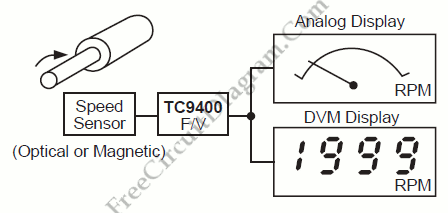# RPM/Speed IndicationOne example of frequency-to-voltage converter application is RPM/Speed indication, converting pulse signal from speed sensor into a linear voltage to drive analog meter. Here is the  block diagram of such speed indication system:Since they measure the number of events per time period, flow rates and revolutions per second are nothing more than frequency signals. This flow and revolution will be converted by optical and magnetic sensors into a digital signal which, in turn, can be converted to a proportional voltage by the use of a frequency-to-voltage (F/V) converter. Then a visual indication of the speed will be given by a simple voltmeter. [Block diagram source: Microchip Application Note]